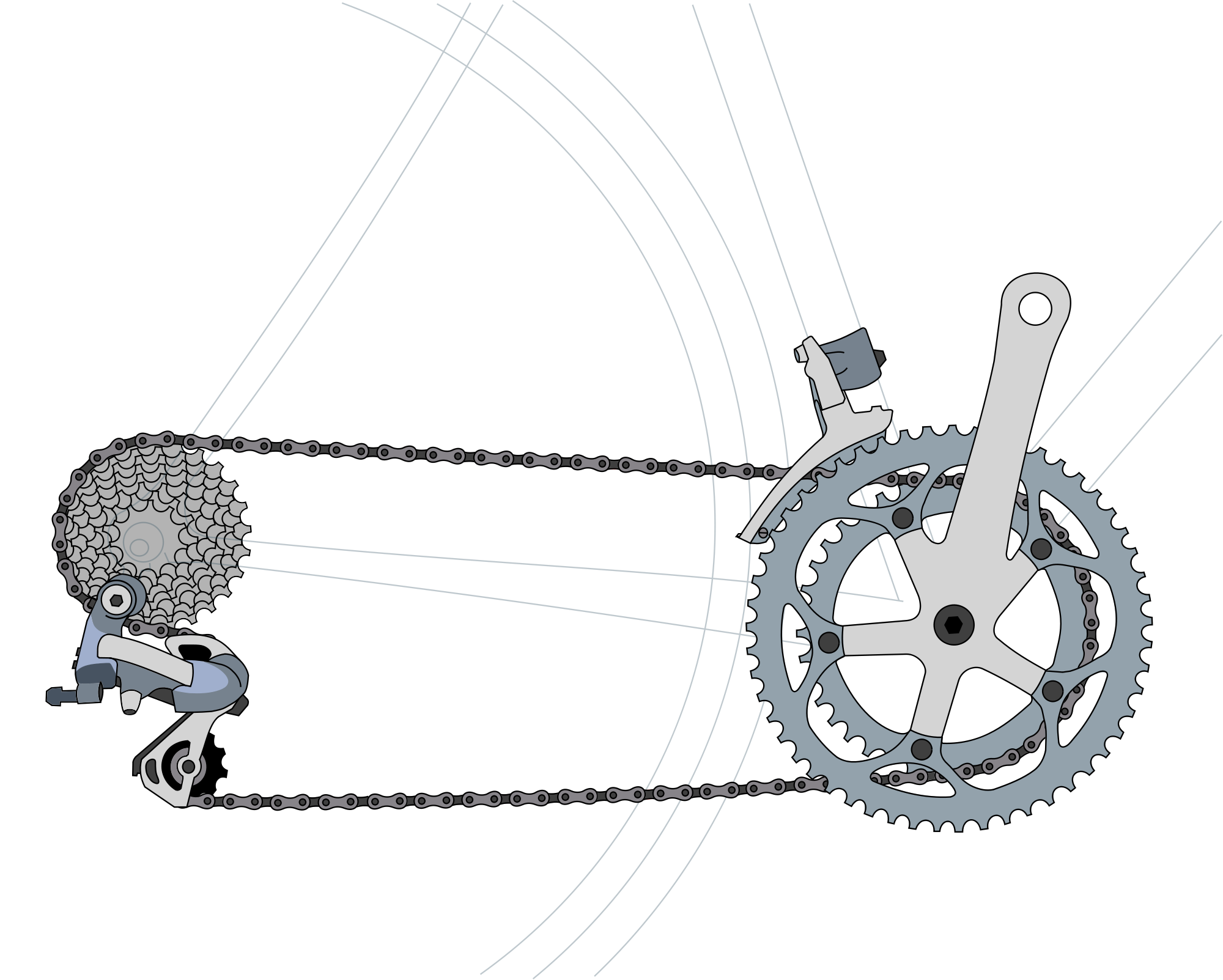# Inference in the FO(C) Modelling Language

Bart Bogaerts, Joost Vennekens, Marc Denecker (KU Leuven)
Jan Van den Bussche (Hasselt University & transnational University of Limburg)

## Goal (FO(C) project)

Develop a good modelling language for causality

## Goal (This paper)

Study inference in FO(C)

## Overview

• FO(C)
• Principles of Causation
• CP-Logic
• C-Log & FO(C)
• Inference
• Background: FO(ID)
• Normal forms for FO(C)
• Relation with FO(ID)
• Complexity of inference in FO(C)

## Principles of Causation

• Universal causation: all events must be triggered by a causal law whose precondition is satisﬁed.
• Sufficient causation: if the precondition for a causal law is satisﬁed, then the event that it triggers must eventually happen.
• Independent causation: which event a causal law triggers is not influenced by other causal laws.

## Cause-effect relations

\begin{align} \mathrm{Turn}(\mathrm{BigGear}) &\leftarrow \mathrm{Pedal}. && (1) \\ \mathrm{Turn}(\mathrm{BigGear}) &\leftarrow \mathrm{Turn}(\mathrm{SmallGear}). && (2)\\ \mathrm{Turn}(\mathrm{SmallGear}) &\leftarrow \mathrm{Turn}(\mathrm{BigGear}). && (3) \end{align}

$\begin{equation}\label{branch} \{\mathrm{P}\} \overset{(1)}{\rightarrow} \{\mathrm{P},\mathrm{T}(\mathrm{B})\} \overset{(3)}{\rightarrow} \{\mathrm{P},\mathrm{T}(\mathrm{B}),\mathrm{T}(\mathrm{S})\} \overset{\text{(2)}}{\rightarrow} \{\mathrm{P},\mathrm{T}(\mathrm{B}),\mathrm{T}(\mathrm{S})\} \end{equation}$## Non-Determinism

$\mathrm{Turn}(\mathrm{SmallGear}) \,\mathbf{Or}\, \mathrm{ChainBreaks} \leftarrow \mathrm{Turn}(\mathrm{BigGear})$

Branching of possibilities

## C-Log

• Extension of CP-Logic
• Motivation: many things are not naturally expressable in CP-Logic
• Expressive language for cause-effect relations
• Non-deterministic choice
• Dynamic set of alternatives
• Object creation
• Arbitrary nesting
• ...

## Dynamic Choice

$\mathbf{Select}\, x[\varphi(x)]: C$

## Lottery

$\mathbf{Select}\, x[Plays(x)]: Rich(x)$

In CP-Logic: only possible if $Plays$ is known statically
$Rich(Alice)\,\mathbf{Or}\,Rich(Bob)\,\mathbf{Or}\,\dots$

## Nesting

Arbitrary nesting of effects

## Lightning

$\left(\mathbf{Select}\, h[House(h)]: (\mathbf{All} \, o[In(o,h)]: Broken(o)) \right)$ $\leftarrow Lightning$

## Object Creation

$\mathbf{New}\, x: C$
Existence of objects governed by causal rules

## Mail Protocol

$\begin{array}{l}\mathbf{All}\, m, t[HitSend(m,t)]: \mathbf{New}\, p:\\ \quad Pack(p) \,\mathbf{And}\, OnCh(p,t)\,\mathbf{And}\, Cont(p,m) \end{array}$

## Summary: syntax

A Causal Effect Expression (CEE) is one of the following:
(if $C$, $C_1$, $C_2$ are CEEs, $\varphi$ a formula, $A$ an atom, $x$ a variable)
• $A$
• $C\leftarrow \varphi$
• $C_1 \,\mathbf{And}\, C_2$
• $C_1 \,\mathbf{Or}\, C_2$
• $\mathbf{All}\, x[\varphi]: C$
• $\mathbf{Select}\, x[\varphi]: C$
• $\mathbf{New}\, x: C$

## Formal semantics

Generalisation of instance-based semantics for D-LP
Based on Approximation Fixpoint Theory

## FO(C)

First-order logic + C-Log

## Overview (this paper)

• Background: FO(ID)
• Normal forms for FO(C)
• Relation with FO(ID)
• Complexity of inference in FO(C)

## FO(ID)

• First-order logic
• Inductive Definitions

## Inductive Definitions

Sets of rules of the form $\forall x: P(\bar{t})\leftarrow \varphi$

## FO(ID) example

$\begin{array}{l} \left\{ \begin{array}{l} \forall x,y: R(x,y) \leftarrow Edge(x,y)\\ \forall x,y: R(x,y) \leftarrow \exists z: R(x,z) \wedge R(z,y) \end{array} \right\}\\ R(Start,End) \end{array}$

## IDP

Knowledge Base System for FO(ID)

Goal: use IDP to perform inference on FO(C)

## Normal Forms And Transformation

• NestNF
• Not "too much" nesting
• Deterministic
• No $\mathbf{Select}$, $\mathbf{Or}$ or  $\mathbf{New}$ expressions
• DefNF
• Of the form $\left\{\begin{array}{l} \mathbf{All}\,\bar{x}_1[\varphi_1]: P_1(\bar{t}_1)\\ \mathbf{All}\,\bar{x}_2[\varphi_2]: P_2(\bar{t}_2)\\\cdots \end{array}\right\}$

## From FO(C) to NestNF

$C$ subexpression of $C_1$
$C_1$ becomes
$\left\{\begin{array}{l} C_1[P(\bar{x})/C] \\\mathbf{All}\, \bar{x}[P(\bar{x})]: C\end{array}\right\}$

## Example

$\mathbf{Select}\, h[House(h)]: (\mathbf{All} \, o[In(o,h)] Broken(o))$ $\leftarrow Lightning$
becomes
$\left\{\begin{array}{l} \mathbf{Select}\, h[House(h)]: Hit(h) \leftarrow Lightning\\ \mathbf{All} \, h[Hit(h)]: \mathbf{All} \, o[In(o,h)] Broken(o)\end{array}\right\}$

## From NestNF to Deterministic

Idea: explicitly represent choices

## Example

$\mathrm{Turn}(\mathrm{SmallGear}) \,\mathbf{Or}\, \mathrm{ChainBreaks} \leftarrow \mathrm{Turn}(\mathrm{BigGear})$
becomes
$\left\{\begin{array}{l}\mathrm{Turn}(\mathrm{SmallGear}) \leftarrow \mathrm{Turn}(\mathrm{BigGear}) \wedge First\\ \mathrm{ChainBreaks} \leftarrow \mathrm{Turn}(\mathrm{BigGear}) \wedge \lnot First\end{array}\right\}$

## Example

$\mathbf{Select}\, x[Plays(x)]: Rich(x)$
becomes
$\begin{array}{l} \left\{\begin{array}{l} \mathbf{All}\, x[Selected(x)]: Rich(x)]\end{array}\right\}\\ \exists_1 x: Selected(x)\\ \forall x: Selected(x)\Rightarrow Play(x)\end{array}$

## From Deterministic to DefNF

Pushing $\mathbf{All}$ through $\mathbf{And}$:

$\mathbf{All}\, x[\varphi]: C_1\,\mathbf{And}\,C_2$
becomes
$\left\{\begin{array}{l} \mathbf{All}\, x[\varphi]: C_1\\ \mathbf{All}\, x[\varphi]: C_2\end{array}\right\}$

NP-complete

## Model Checking

NP-complete

FO(C) theory $T$: transform to $\exists \bar P: T'$, where $T'$ is an FO(ID) theory
Model checking of FO(C) = Model checking of $\exists SO$

## (Unbounded) Endogenous Model Generation

Given: domain + interpretation for exogenous symbols
Find: model with larger domain (all new elements created by $\mathbf{New}$)
Can decide every decidable class of finite structures

## Implementation

Prototype of transformation, model expansion and model checking in IDP

## References

( , , and )
• Inference in the FO(C) Modelling Language
• European Conference on Artificial Intelligence (ECAI), 2014.
• 15th International Workshop on Non-Monotonic Reasoning 2014 .
• FO(C) and Related Modelling Paradigms 15th International Workshop on Non-Monotonic Reasoning, 2014.

• FO(C): A Knowledge Representation Language of Causality International Conference of Logic Programming (ICLP), (Technical communication), 2014.